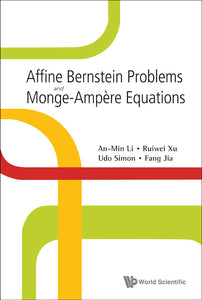# Affine Bernstein Problems And Monge-ampere Equations

An-min Li

Format: Print Book

ISBN: 9789812814166

• SGD 117.70
Unit price per
Tax included.

In this monograph, the interplay between geometry and partial differential equations (PDEs) is of particular interest. It gives a selfcontained introduction to research in the last decade concerning global problems in the theory of submanifolds, leading to some types of Monge-Ampère equations.From the methodical point of view, it introduces the solution of certain Monge-Ampère equations via geometric modeling techniques. Here geometric modeling means the appropriate choice of a normalization and its induced geometry on a hypersurface defined by a local strongly convex global graph. For a better understanding of the modeling techniques, the authors give a selfcontained summary of relative hypersurface theory, they derive important PDEs (e.g. affine spheres, affine maximal surfaces, and the affine constant mean curvature equation). Concerning modeling techniques, emphasis is on carefully structured proofs and exemplary comparisons between different modelings.

Format: Hardcover
No of Pages: 192
Imprint: World Scientific
Publication date: 20100428